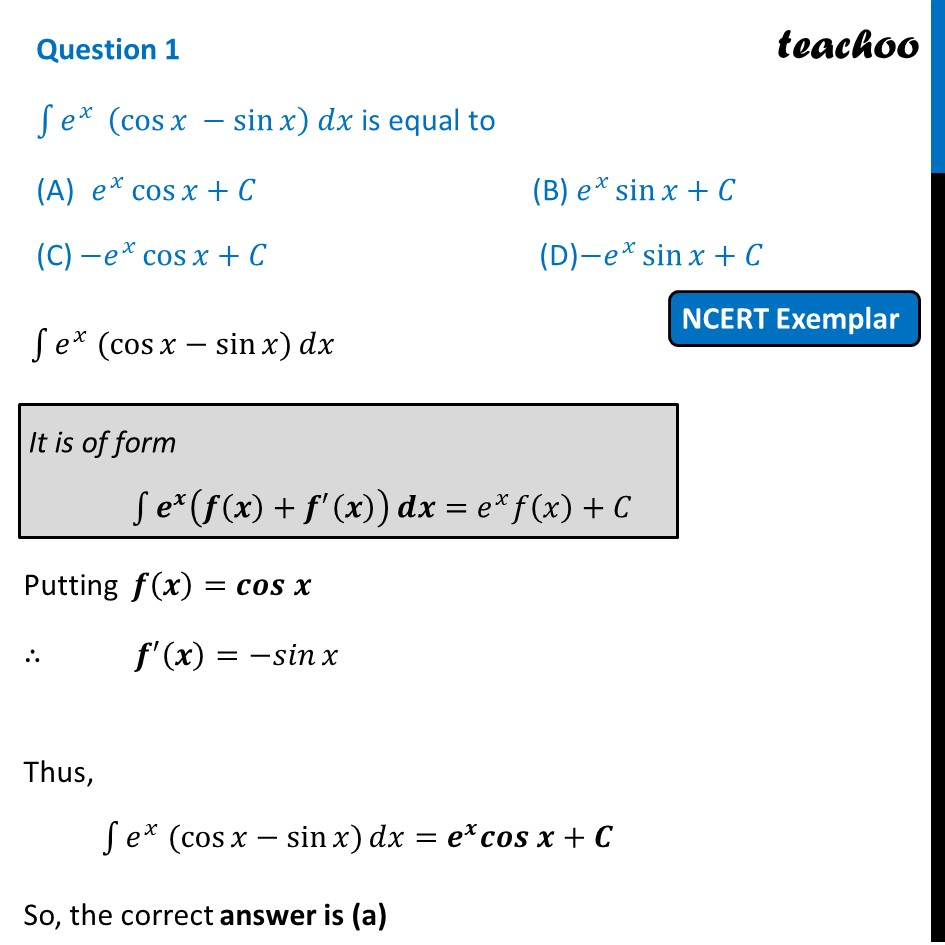NCERT Exemplar MCQ

Chapter 7 Class 12 Integrals
Serial order wise

## (D) -e^x  sin⁡x + C

This question is similar to Ex 7.6, 16 - Chapter 7 Class 12 - IntegralsLearn in your speed, with individual attention - Teachoo Maths 1-on-1 Class

### Transcript

Question 1 ∫1▒𝑒^𝑥 (cos⁡𝑥 −sin⁡𝑥 ) 𝑑𝑥 is equal to 𝑒^𝑥 cos⁡𝑥+𝐶 (B) 𝑒^𝑥 sin⁡𝑥+𝐶 (C) 〖−𝑒〗^𝑥 cos⁡𝑥+𝐶 (D)〖−𝑒〗^𝑥 sin⁡𝑥+𝐶 ∫1▒〖𝑒^𝑥 (cos⁡𝑥−sin⁡𝑥)〗 𝑑𝑥 Putting 𝒇(𝒙)=𝒄𝒐𝒔 𝒙" " ∴ 𝒇^′ (𝒙)=〖−𝑠𝑖𝑛〗⁡𝑥 Thus, ∫1▒〖𝑒^𝑥 (cos⁡𝑥−sin⁡𝑥)〗 𝑑𝑥=𝒆^𝒙 𝒄𝒐𝒔 𝒙+𝑪 So, the correct answer is (a)